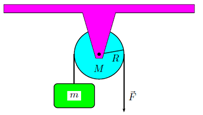# Problem: A pulley of mass M and radius R pivots freely about its center with Icm = kM R  2, where k is some numerical constant. A string is attached to a mass m and run over the pulley as in the sketch. If a downward force F is applied by your hand to the string, find a, the acceleration of the block in terms of F, m, M, k and g only. Take upward to be positive. 1. a = F + mg/ (k + 1) m 2. a = F - mg/ kM - m 3. a = F - mg/ (k + 1) M 4. a = F - mg/ (kM + m) 5. a = F - mg/ kM - m 6. a = F - mg/ (k + 1) m 7. a = F - mg/ (k - 1) M 8. a = F + mg/ (k + 1) M 9. a = F - mg/ (k - 1) m 10. a = F + mg/ kM + m

###### FREE Expert Solution
87% (329 ratings)
###### Problem Details

A pulley of mass M and radius R pivots freely about its center with Icm = kM R  2, where k is some numerical constant. A string is attached to a mass m and run over the pulley as in the sketch. If a downward force F is applied by your hand to the string, find a, the acceleration of the block in terms of F, m, M, k and g only. Take upward to be positive.

1. a = F + mg/ (k + 1) m

2. a = F - mg/ kM - m

3. a = F - mg/ (k + 1) M

4. a = F - mg/ (kM + m)

5. a = F - mg/ kM - m

6. a = F - mg/ (k + 1) m

7. a = F - mg/ (k - 1) M

8. a = F + mg/ (k + 1) M

9. a = F - mg/ (k - 1) m

10. a = F + mg/ kM + m